Olympiad Test: Basic Geometrical Ideas

# Olympiad Test: Basic Geometrical Ideas

Test Description

## 20 Questions MCQ Test Mathematics (Maths) Class 6 | Olympiad Test: Basic Geometrical Ideas

Olympiad Test: Basic Geometrical Ideas for Class 6 2022 is part of Mathematics (Maths) Class 6 preparation. The Olympiad Test: Basic Geometrical Ideas questions and answers have been prepared according to the Class 6 exam syllabus.The Olympiad Test: Basic Geometrical Ideas MCQs are made for Class 6 2022 Exam. Find important definitions, questions, notes, meanings, examples, exercises, MCQs and online tests for Olympiad Test: Basic Geometrical Ideas below.
Solutions of Olympiad Test: Basic Geometrical Ideas questions in English are available as part of our Mathematics (Maths) Class 6 for Class 6 & Olympiad Test: Basic Geometrical Ideas solutions in Hindi for Mathematics (Maths) Class 6 course. Download more important topics, notes, lectures and mock test series for Class 6 Exam by signing up for free. Attempt Olympiad Test: Basic Geometrical Ideas | 20 questions in 20 minutes | Mock test for Class 6 preparation | Free important questions MCQ to study Mathematics (Maths) Class 6 for Class 6 Exam | Download free PDF with solutions
 1 Crore+ students have signed up on EduRev. Have you?
Olympiad Test: Basic Geometrical Ideas - Question 1

### What is the simplest of all geometrical figures which has no size but has a position?

Olympiad Test: Basic Geometrical Ideas - Question 2

### What is a set of points which extend infinitely in both directions called?

Olympiad Test: Basic Geometrical Ideas - Question 3

### Name the set of points which is a part of a line with two end points.

Olympiad Test: Basic Geometrical Ideas - Question 4

How is a line PQ symbolically written?

Detailed Solution for Olympiad Test: Basic Geometrical Ideas - Question 4

The symbol of a line is ↔.
Hence, a line PQ is symbolically written as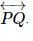Olympiad Test: Basic Geometrical Ideas - Question 5

How do you write a line segment AB symbolically?

Olympiad Test: Basic Geometrical Ideas - Question 6

What is the symbolic representation of a ray OP?

Olympiad Test: Basic Geometrical Ideas - Question 7

What are used to represent points?

Olympiad Test: Basic Geometrical Ideas - Question 8

What is the number of end points of a line?

Olympiad Test: Basic Geometrical Ideas - Question 9

What is a set of points extending infinitely in all directions on the same flat surface called?

Olympiad Test: Basic Geometrical Ideas - Question 10

How many lines can be drawn passing through a given point?

Olympiad Test: Basic Geometrical Ideas - Question 11

How many lines can you draw joining two distinct points in a plane?

Detailed Solution for Olympiad Test: Basic Geometrical Ideas - Question 11

Only one line can be drawn passing through two given points.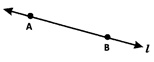Olympiad Test: Basic Geometrical Ideas - Question 12

Three points P, Q and R are said to be collinear. Where do they lie?

Detailed Solution for Olympiad Test: Basic Geometrical Ideas - Question 12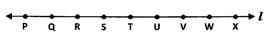The points P, Q, R, S, T ... lying on line ℓ are said to be collinear.
NOTE: Infinite number of points lying on same line are considered as collinear.

Olympiad Test: Basic Geometrical Ideas - Question 13

Four lines p, q, r and s are said to be concurrent. What do they pass through?

Detailed Solution for Olympiad Test: Basic Geometrical Ideas - Question 13

If the lines p, q, r and s are said to be concurrent they pass through the same point.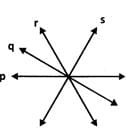Olympiad Test: Basic Geometrical Ideas - Question 14

In the given figure what is point P called?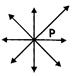Olympiad Test: Basic Geometrical Ideas - Question 15

In the given figure, what are lines l, m and n called?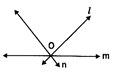Olympiad Test: Basic Geometrical Ideas - Question 16

In the given figure, what are points P, O and Q called?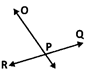Olympiad Test: Basic Geometrical Ideas - Question 17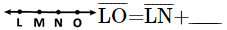Olympiad Test: Basic Geometrical Ideas - Question 18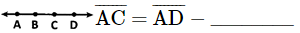Olympiad Test: Basic Geometrical Ideas - Question 19

Which instrument is used to compare two line segments?

Detailed Solution for Olympiad Test: Basic Geometrical Ideas - Question 19

A divider is used to compare line segments.

Olympiad Test: Basic Geometrical Ideas - Question 20

Which of these instruments are used to construct a line segment?

## Mathematics (Maths) Class 6

228 videos|275 docs|43 tests
 Use Code STAYHOME200 and get INR 200 additional OFF Use Coupon Code
Information about Olympiad Test: Basic Geometrical Ideas Page
In this test you can find the Exam questions for Olympiad Test: Basic Geometrical Ideas solved & explained in the simplest way possible. Besides giving Questions and answers for Olympiad Test: Basic Geometrical Ideas, EduRev gives you an ample number of Online tests for practice

## Mathematics (Maths) Class 6

228 videos|275 docs|43 tests